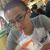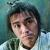寻找一个数组重组的算法.

【推荐阅读】滴滴出行的数据架构和信息流处理思路解析->>>\$a = [1,2,3,4,5,6,7,8,9]

\$b = [1,2] \$b = [1,3] \$b = [1,4] \$b = [1,5]

000
f
<?php
\$array = array(1,2,3,4,5,6,7,8,9);
echo reform(\$array,5);
//组合数公式： Cmn = Pmn / Pmm = n(n-1)(n-2)...(n-m+1)/m! = n!/m!/(n-m)!
function reform(\$array=null,\$length=0){
if(!\$length || !\$array) return 0;
if(count(\$array) < \$length) return 0;
\$total = count(\$array);
\$n = \$m = 1;
for(\$s=0;\$s<\$length;++\$s){
\$n = \$n * (\$total-\$s);
\$m = \$m * (\$s+1);
}
\$i = \$n/\$m;
return \$i;
}